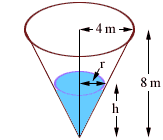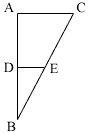Quandaries and Queries Adrienne secondary (12th grade) student Hello, I am having difficulty figuring out this problem.� Thank you in advance, any help is much appreciated. Water is poured into a tank in the shape of an inverted right circular cone.� The height of the tank is 8 m and its radius at the top is 4 m. a. Draw and label a picture to represent this situation.� (I know how to do this) b. Identify all variable quantities. (h = 8m, r = 4m) c. Find an equation that relates the variable quantities, and reduce the number of variable quantities to two. I was thinking about the equation V = 1/3 pi r2 h, which is the Volume of a cone, but I am stumped as to how I am supposed to "reduce the number of variable quantities to two." Can you point me in the right direction? Thank you, Adrienne Hi Adrienne, Here is my diagram.The important variables are r and h, the height and radius of the water in the cone. Then, as you said, the volume of the water in the cone is V = 1/3 pi r2 h cubic meters. To reduce the number of variables look back at the diagram. I see a triangle, which I have labeled ABC, with |AB| = 8 meters and |CA| = 4 meters.Inside is the triangle DBE with |DB| = h meters and |ED| = r meters. The triangles ABC and DBE are similar so  |AB|/|CA| = |DB|/|ED| That is  8/4 = h/r so h = 2 r. If you substitute this into the equation for the volume of the water in the cone you will have an expression with the only variables being V and r. Chers, Penny Go to Math Central